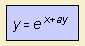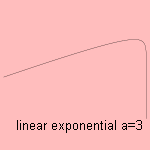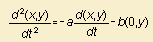# linear exponential

## exponential

last updated: 2005-11-11The curve has a linear form of y and x in the exponent, also to be written as:

y = ln x + a x

The curve emerges for a ball thrown away when taken account of air friction, proportional to the speed 1). Changing the equation to y = ln (-x) + x shows the thrown ball curves.
Without that friction, the curve is a parabola, for which reason you can call the curve a braked parabola.notes

1) This leads to the following differential equation: